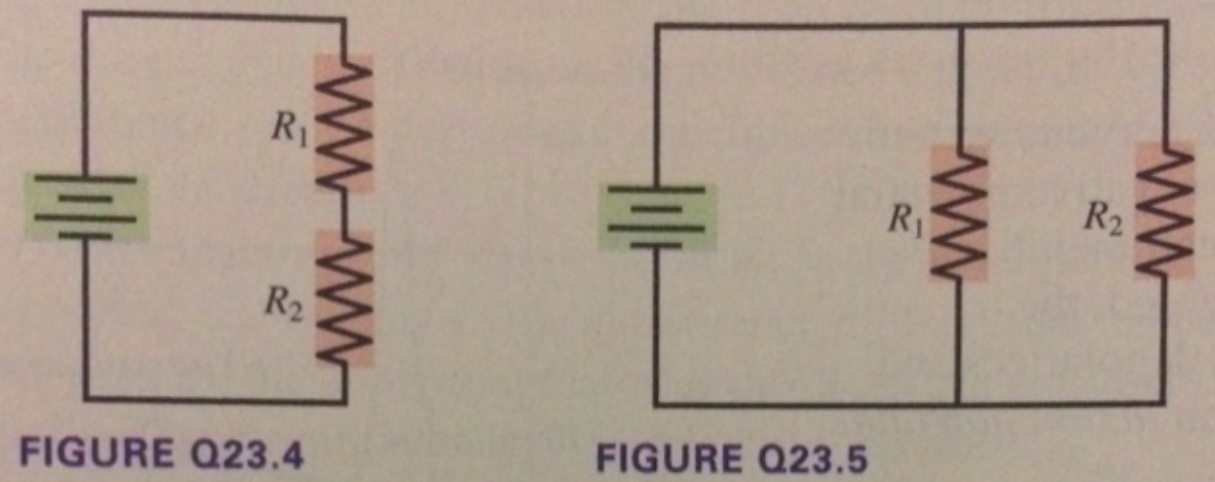# Problem: 1. The circuit in Figure Q23.4 has two resistors, with R1 &gt; R2. Which resistor dissipates the larger amount of power? Explain.2. The circuit in Figure Q23.5 has a battery and two resistors, with R1 &gt; R2. Which resistor dissipates the larger amount of power? Explain.

###### FREE Expert Solution

Power in circuits:

$\overline{){\mathbf{P}}{\mathbf{=}}{{\mathbf{i}}}^{{\mathbf{2}}}{\mathbf{R}}}$, where i is the current, and R is the resistance.

84% (103 ratings)###### Problem Details

1. The circuit in Figure Q23.4 has two resistors, with R1 > R2. Which resistor dissipates the larger amount of power? Explain.

2. The circuit in Figure Q23.5 has a battery and two resistors, with R1 > R2. Which resistor dissipates the larger amount of power? Explain.